﻿ Optimal Packing# Optimal Packing

It all started with a puzzle …

In which of these three pictures do the circles cover the highest percentage of the square?Some people see it right away, others work it out on paper. It's not really a trick question, it's an interesting result. The answer is, of course, that all three are the same. Do you see it? Let's prove it with math.

Let's define the square to have side length of one unit. The Area of each square is, therefore, one unit squared.

Now let's find the area of the circles:

One circle - The area of the circle is πr2 = π(1/2)2 = π/4

Four circles - The area of the circles is 4(πr2) = 4(π(1/4)2) = 4(π(1/16)) = π/4

Nine circles - The area of the circles is 9(πr2) = 9(π(1/6)2) = 9(π(1/36)) = π/4

I think you can see it does not matter how many square-packed tessellated circles we put into the unit square, the answer will always be π/4. When there are n circles on an edge, there are a total of n2 circles, each of area π(1/2n)2.You can see why this makes sense. Each of the more complex versions of the shape are self-similar to the fundamental shape. Whatever the ratio of the square to the circle, the (2x2) is made entirely of building blocks that are the same ratio. The ratio stays the same. And so on …
 An other interesting consequence of this is that the two shaded shapes here (the quarter circle and the circle with half the radius) have the same area.### Square Packing vs. Hexagonal packing

Square packing achieves a packing ration of π/4 (approx 78.54%), which is pretty good. It isn't, however, the only way to tightly tessellate circles in a plane. By staggering every other row, and nesting them tightly together we create an arrangement that packs more efficiently. This arrangement is called hexagonal packing with an efficiency of approx 90.69%Square Packing Hexagonal Packing

### Packing Boxes

Hexagonal packing is a much more efficient user of space; if we had an infinite plane to pack, but reality adds constraints. Imagine we needed to pack tubes of cylindrical candy into a shipping carton (or pack cans of soda into a tray).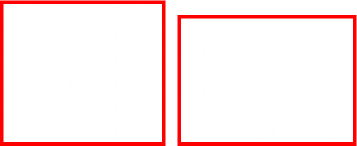Square packing does not suffer from edge-effects. In hexagonal packing, every other row has to miss out of part of a circle from either end. This creates a big waste of space. When the size of the circles to be packed is large (compared to the box), these additional voids are significantly large enough to over-rule the higher packing density. However, as the circles get smaller (or box gets bigger!), even with these extra voids, the closer packing of the rest of the circles results in a better fit.

In the example above, the box packing the circles on the left is taller than that on the right, but the one on the right is a little bit wider. They both contain the same number of circles. Which is the larger area? There's not much in it, but the area (length x width) of the box on the right is just that little bit smaller than that on the left.

### Further down the rabbit hole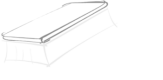There's an interesting study in mathematics, and this is to find out the most efficient way to pack n circles into a square. For small numbers of circles, the results are obvious, have obvious symmetry, and have been mathematically proven to be the optimal. Above approximately twenty, however, the optimal answers have not been proven, or even known/confirmed. Currently known best solutions have been determined by various approximating algorithms (simulated annealing, and randomly 'jiggling' the circles whilst trying to reduce the wall widths like a trash compactor on the Death Star).
 Below are solutions for the arrangements of the first twenty sets of circles. For each number of circles, I've shown just one of the layouts (there are trivial reflections and rotations for all the solutions), and sometimes canonical forms. For each I've shown the packing efficiency η as a percentage, and the radius r that the circle would need to be to fit this configuration into a unit square. If, instead, you want the size of the square that is needed to contain that number of unit-radius circles, simply take the reciprocal of radius value. (This is the solution to what is the smallest piece of square pastry you would need is order to be able to cut out n unit-radius circular pie crusts with the smallest amount of waste).Image: Drift Words
 n=1 n=2 n=3 n=4 n=5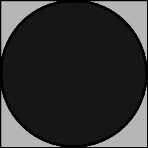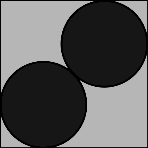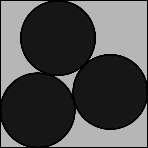η = 78.54%    r = 0.5000 η = 53.90%    r = 0.2929 η = 60.96%    r = 0.2543 η = 78.54%    r = 0.2500 η = 67.38%    r = 0.2071 The first few solutions should surprise nobody. These all look the configurations we'd expect. When n=4 we can see the first (non-trivial) appearance of the square packing configuration. n=6 n=7 n=8 n=9 n=10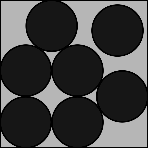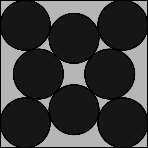η = 66.40%    r = 0.1877 η = 66.93%    r = 0.1745 η = 73.10%    r = 0.1705 η = 78.54%    r = 0.1667 η = 69.00%    r = 0.1482 The shape for n=6 might be a little of a surprise, and for n=7 there is a 'loose' circle that rattles around. Symmetry returns for n=8 and n=9, and n=10 almost looks random! n=11 n=12 n=13 n=14 n=15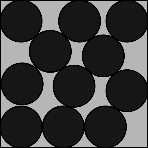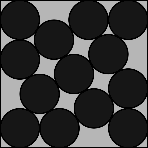η = 70.07%    r = 0.1424 η = 73.85%    r = 0.1400 η = 73.33%    r = 0.1340 η = 73.57%    r = 0.1293 η = 76.21%    r = 0.1272 n=11 has a couple of loose circles, and though it look like a hexagonal packing, n=12 has bigger gaps between some adjacent circles. n=13 and n=14 also both have loose circles. n=16 n=17 n=18 n=19 n=20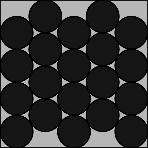η = 78.54%    r = 0.1250 η = 73.36%    r = 0.1172 η = 75.47%    r = 0.1155 η = 75.23%    r = 0.1123 η = 77.95%    r = 0.1114

Square packing returns for n=16. Square packing is still the optimal for the next perfect squares of n=25 (5x5), and n=36 (6x6), but by the time we get to n=49 (7x7), it is no longer the optimal.

As mentioned above, hexagonal packing is, theoretically, the most efficient packing scheme, but suffers from edge effects. As the circles get smaller, and their number increases, these edge effects become less significantly. By n=49, a distorted square pack (squashed and sheared slightly towards a hexagonal packing is the most efficient packing) becomes more efficient. It's not very pretty, but it takes less space. At n increases, the patterns get closer to hexagonal packing.

 n=49 n=64 n=81 n=144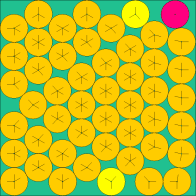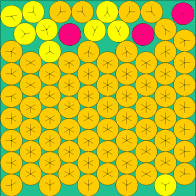The above images come from this (very excellent) web site of the current known optimal solutions.

### Graph of efficiency

Here is a graph of how the optimal efficiency changes with n:The red reference line shows the 78.54% percent that square packing achieves.

• For n=1,4,9,16,25 the packing on these perfect squares, by definition, meets the red line.

• From above, by the perfect square n=49 (7x7), we are already more efficient than square packing.

• The first time that a packing achieves a higher density than square packing is when n=30

• Past n=38, every packing efficiency is higher than square packing efficiency of 78.54%

• There are spikes at n=39, n=52, n=68, n=86 and n=99. These look very close to hexagonal packs when you look at them

## Packing in three dimensionsThe principles of packing circles into squares can be extended into three dimensions to cover the concept of packing spherical balls into cubic boxes. As with 2D, the optimal packing for a single layer is a hexagonal arrangement, with each sphere being surrounded and touching six others in the plane. On top of this, we can layer another carpet of hexagonal packed balls, aligned so that the centers of the new layer lay in the crotch of the layer below. This will give us a 'two layer' cake. We can label the layers A and B to differentiate.

Ontop of these two layers, we have two choices. We can make our third layer be a duplicated of the first layer, so that the two layers alternate ABABAB, or we can make the third layer appear over the top of the void in the first layer that has not been covered yet. This three layer pattern then repeats ABCABC. These two variants are shown below:

 Hexagonal Close Pack Face Centered Close PackABABAB ABCABC

Both of these result in optimal packing (you can see there should be no difference between them, the C layer is just like the A layer, but rotated 180°). These structures are studied by chemists as they represent the arrangement of atoms in some crystalline elements.

The ABABAB format is given the name Hexagonal Close Packing, and the ABCABC format is given the name Face Centered Cubic Close Packing (reflecting the fact that the eight points on adjacent layers two apart make the corners of a 'cube' with an additional sphere in the middle).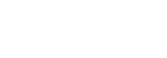In both arrangements, each sphere touches six spheres in the same layer, and three from the layer above, and three from the layer below. Every sphere touches twelve others. The efficiency of a close packing is just over 74%

Hexagonal close pack is the structure seen in crystals of Be, Co, Mg, and Zn (and also He, if you get it really, really cold).

Face centered cubic (also called cubic close pack) is the structure see in elements such as Ag, Al, Au, Ca, Co, Cu, Ni, Pb, and Pt (also all the rare gasses, except He, if the temperature is lowered enough to make them solid).

### Guess the number of bubble gums

If you look about, you'll see plenty of hexagonal close packing around you. If you tip marbles into a jar, they'll land into something like a hexagonal close pack.

A pyramid of stacked canon balls is stored as a hexagonal close pack.Image Let Ideas CompeteImage Nigel Munoz

Now that you know that hexagonal packing is approx 75% efficient, next time there is a 'guess the number of bubblegums in the machine' competition, knowing the diameter of the container, the diameter of a gumball, and a little high school math, you can make a much more educated guess. If you win, be sure to share.

You can find a complete list of all the articles here.Click here to receive email alerts on new articles.